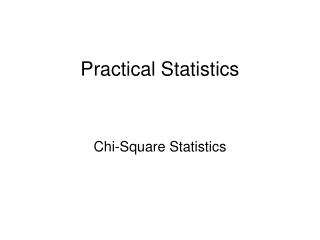DownloadDownload PresentationPractical Statistics

# Practical Statistics

Télécharger la présentation## Practical Statistics

- - - - - - - - - - - - - - - - - - - - - - - - - - - E N D - - - - - - - - - - - - - - - - - - - - - - - - - - -
##### Presentation Transcript

1. Practical Statistics Chi-Square Statistics

2. There are six statistics that will • answer90% of all questions! • Descriptive • Chi-square • Z-tests • Comparison of Means • Correlation • Regression

3. Chi-square: Chi-square is a simple test for counts…..

4. Chi-square: Chi-square is a simple test for counts….. Which means: nominal data and… if some cases… Ordinal data

5. Chi-square: • There are three types: • Test for population variance • Test of “goodness-of-fit” • Contingency table analysis

6. Chi-square: • There are three types: • Test for population variance

7. Chi-square: • There are three types: • Test for population variance • Test of “goodness-of-fit” Where o = frequency of actual observation, and e = frequency you expected to find

8. According to marketing research, the clientele of a Monkey Shine Restaurant is made up of 30% Western businessmen, 30% women who stop in while shopping, 30% Chinese business men, and 10% tourists. A random sample of 600 customers at the Kowloon Monkey Shine found 150 Western businessmen, 190 Chinese businessmen, 100 tourists, and 65 women who were shopping. Is the clientele at this establishment different than the norm of the this company?

9. = 5.00 + 0.56 + 2.22 + 26.67 = 34.45 With (4-1) degrees of freedom

10. The chi-square distribution is highly skewed and dependent upon how many degrees of freedom (df) a problems has.

11. The chi-square for the restaurant problem was: Chi-square = 34.45, df = 3 By looking in a table, the critical value of Chi-square with df = 3 is 7.82. The probability that the researched frequency equals the frequency found in the MR project was p < .001. http://www.fourmilab.ch/rpkp/experiments/analysis/chiCalc.html

12. By looking at the analysis, it is obvious that the largest contribution to chi-square came from the tourists. = 5.00 + 0.56 + 2.22 + 26.67 = 34.45 df = 3 Hence, the Kowloon property is attracting more tourist than what would be expected at the Monkey Shine.

13. Chi-square: • There are three types: • Test for population variance • Test of “goodness-of-fit” • Contingency table analysis Where o = frequency of actual observation, and e = frequency you expected to find

14. A contingency table is a table with numbers grouped by frequency.

15. A contingency table is a table with numbers grouped by frequency. There are three groups: brand loyal customers, regular buyers, and occasional buyers. Each is asked if they like the taste of new product over the old. They answer with a “yes” or a “no.”

16. A contingency table would look like this:

17. A contingency table is a table with numbers grouped by frequency. All the numbers in the table are “observed” frequencies (o). So, what are the expected values?

18. The expected values (e) would be a random distribution of frequencies.

19. The expected values(e) would be a random distribution of frequencies. These can be calculated by multiplying the row frequency by the column frequency and dividing by the total number of observations.

20. For example, the expected values (e) of “loyal” And “yes” would be (150 X 90)/270 = 50

21. For example, the expected values (e) of “regular” And “no” would be (120 X 100)/270 = 44.4

22. The chi-square value is calculated for every cell, and then summed over all the cells.

23. The chi-square value is calculated for every cell: For Cell A: (50-50)^2/50 = 0 For Cell D: (40-44.4)^/44.4 = 0.44

24. The chi-square value is calculated for every cell:

25. The chi-square value is calculated for every cell: Chi-square = 0 + 0 + .35 + .44 + .44 + .54 = 1.77 The df = (r-1)(c-1) = 1 X 2 = 2

26. A chi-square with a df = 2 has a critical value of 5.99, this chi-square = 1.77, so the results are nonsignificant. http://www.fourmilab.ch/rpkp/experiments/analysis/chiCalc.html The probability = 0.4127. This means that the distribution is random, and there is no association between customer type And taste preference.

27. A chi-square with a df = 2 has a critical value of 5.99, this chi-square = 1.77, so the results are nonsignificant. This means that the distribution is random, and there is no association between customer type And taste preference. Note: This type of chi-square is a test of association using nothing but counts (frequency); VERY useful in business research.